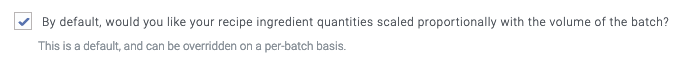# How does Breww scale the ingredients on batches (and how does this change with multi-turn batches)?

## Quantity calculation method

Breww follows the following steps when determining how to adjust the ingredient quantities added to a batch from a recipe.

1. First, Breww checks whether you’ve chosen to Scale recipe quantities to match batch volume.
2. If so, Breww calculates the ratio between the recipe’s volume and the batch’s planned volume and multiplies all ingredient additions by this ratio to get the quantity to add for each ingredient addition.
3. Next, Breww checks if this batch is a multi-turn batch.
4. If it is, Breww goes through all of the ingredient additions and checks whether they are added during a stage that Repeats per turn.
5. If they are, Breww gets the scaled ingredient quantity calculated earlier and splits it proportionally between each turn.

When you’re making a recipe and adding ingredients, you need to enter the full amount to use in total on the recipe. There is no need to anticipate how many turns will be done later and try to adjust for this as Breww will do this for you. For example, if in the recipe you enter 100kg of an ingredient, and then make a two turn batch, Breww will prompt you for 50kg per turn (assuming the batch volume is the same as the recipe volume).

graph TD A[Is auto-scaling enabled?] A --> |Yes|SY[Calculate scaling ratio<br>between recipe and<br>batch planned quantity,<br>then adjust quantities<br>by scaling ratio.] A --> |No|SN[Use the quantity on the recipe<br>without changes.] SY --> MT[Is this the recipe stage a multi-turn stage?] MT --> |Yes|MTY[Divide the quantity by the number of turns<br>and require this quantity per turn.<br>The total quantity on the batch isn't changed<br>by multi-turn being enabled or disabled, it's just<br>split between all the turns evenly.] MT --> |No|MTN[Non-multi-turn stages, so<br>simply use the quantity.] SN --> MT

## Simple example

Let’s assume you entered 330kg of a malt on a multi-turn recipe step and gave your recipe a batch size of 500 Litres then:

• If you brew a 500L batch with one turn, that turn will need 330kg of the malt
• If you brew a 500L batch with two turns, each turn will need 160kg of the malt (but still 330kg in total)
• If you brew a 1,000L batch with one turn and enable scaling, that turn will need 660kg of the malt
• If you brew a 1,000L batch with two turns and enable scaling, each turn will need 330kg of the malt (but still 660kg in total)
• If you brew a 1,000L batch with one turn and disable scaling, that turn will need 330kg of the malt
• If you brew a 1,000L batch with two turns and disable scaling, each turn will need 165kg of the malt (but still 330kg in total)

As you can see, the number of turns doesn’t impact the total amount of ingredients used, just the amount per turn (to reach the same grand total).

The rules to remember are:

• Scaling being enabled will increase (or decrease) the total amount of stock required for the batch from what was on the recipe.
• If scaling is disabled, then the total amount of stock required will be the quantity entered into the recipe, regardless of how big the batch is.
• Once the total quantity has been determined, this quantity will be divided by the number of turns to get the amount used per turn. The number of turns will never impact the total quantity of the ingredient that will be used for the batch, it will just be split over the turns.

## Complex example

As an example, we’ll use the following simple recipe.

Recipe volume: 1,000 L

Stages:

• Mash (Repeats per turn)
• 100 kg x Maris Otter
• Boil (Repeats per turn)
• 20 kg x Citra
• Dry hop
• 15 kg x Amarillo

Let’s say we are brewing a 2,000 L batch of the above recipe over two turns and have chosen to scale the recipe up to 2,000 L. First, Breww calculates the scaling ratio: 2,000 L / 1,000 L = 2. Next, Breww scales the total ingredient quantities by this ratio. So, 100 kg of Maris Otter in the Mash would be scaled to 200 kg, 20 kg of Citra in the boil would be scaled to 40 kg, and 15 kg of Amarillo would be scaled to 30 kg.

Next, Breww then looks at each ingredient addition and whether it is part of a stage that Repeats per turn. As we are brewing this recipe over two turns, in our Mash and Boil, the quantity of each ingredient addition will be split proportionally amongst the turns, whilst our Dry hop additions won’t be. So Breww will add 100 kg of the total 200 kg of Maris Otter, and 20 kg of the total 40 kg of Citra, to each turn. The Amarillo is used in the Dry hop, which isn’t a Repeats per turn stage, so this stage only occurs once and has the entire 30 kg calculated earlier.

This method means that the ratios of the ingredients used remain the same between the stages that Repeats per turn and those that don’t. In the example above, the ratio is always 100 kg of Maris Otter used to 15 kg of Amarillo. Whereas if Breww didn’t evenly split the total Maris Otter proportionally per turn, then a two-turn batch would have 200 kg of Maris Otter to 15 kg of Amarillo, and a three-turn batch 300 kg to 15 kg etc.